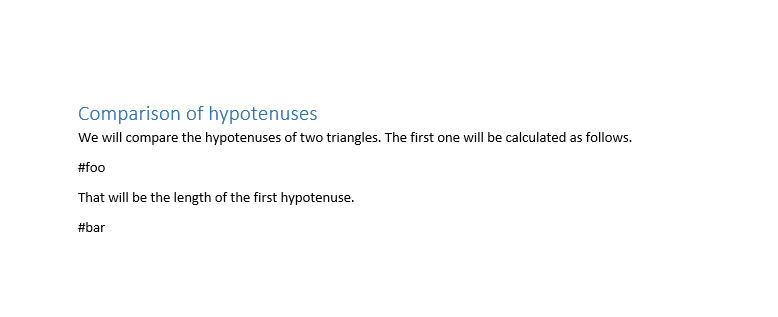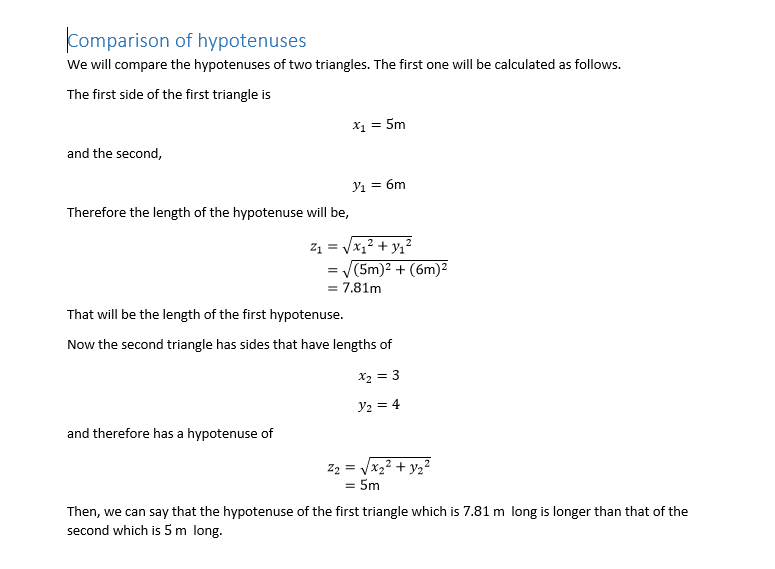# docal Release 3.0.4

Inject calculations into Word and LaTeX documents with ease!

Keywords
docal, math, latex, word, python, engineering, python-library, equation, latex-document, calculations, scientific-reports
MIT
Install
``` pip install docal==3.0.4 ```

# docalInject Python calculations into Word and LaTeX documents with ease!

docal is a tool that can be used to send calculations that are written in python to Word or LaTeX documents. It evaluates equations in a separate python script from the document and replaces hashtags in the document that indicate where the calculations should be with the results of the evaluation. It comes with a powerful python expression to LaTeX converter built-in, so it converts the calculations and their results to their appropriate LaTeX forms before sending them, which makes it ideal to make academic and scientific reports.

## Installation

### Requirements

A basic understanding of Python in general is necessary to have a smooth experience here (although you will do fine even if you don't).If you want to work with a little more advanvced stuff, like arrays and matrices, more knowledge about python is necessary.

It must be obvious by now but you should have Python installed on your system. You can check that by opening your shell (see above) and typing the command `python` and hitting Enter. If it writes the version number and other info about your python installation, you already have it installed. If the version number starts with 2, you should probably install python 3 (the latest). If you have python 3 or above, you're good to go. If either you don't have Python 3 or you don't have Python at all, you should go to Python's homepage and install it, making sure to check the box "add python to path" during installation.

If you want to work with word documents, you should have Pandoc installed on your system (and in your PATH). Because docal internally only works with tex files and when a word file is given, it internally converts it to tex, modifies it and converts it back to word, using pandoc.

### Install

To install this package, (after making sure that you have a working internet connection) type the following command and hit Enter.

`pip install docal`

Or from the source:

`pip install .`

## Usage

### Typical workflow

• The user writes the static parts of the document as usual (Word or Latex) but leaving sort of unique hashtags (#tagname) for the calculation parts (double hash signs for Wrod).

• The calculations are written on a separate text file with any text editor (Notepad included) and saved next to the document file. For the syntax of the calculation file, see below. But it's just a python script with comments.

• The tool is invoked with the following command:

`docal [calculation-file] -i [input-file] -o [output-file]`

so for example,

`docal calcs.py -i document.tex -o document-out.tex`
• Then voila! what is needed is done. The output file can be used normally.

### Syntax

The syntax is simple. Just write the equations one line at a time. What you write in the syntax is a valid Python file, (it is just a script with a lot of assignments and comments).

#### Comments that take whole lines

These comments are converted to paragraphs or equations, depending on what comes immediately after the hash sign. If the hash sign is followed by a single dollar sign (\$), the rest of that line is expected to be a python equation, and will be converted to an inline LaTeX equation. If what comes after the dollar sign is two dollar signs, the rest of that line will be converted to a displayed (block) equation in the document. Remember, these equations are still in comments, and thus do not do anything except appear as equations. If the hash sign is followed by just running text, it is converted to a paragraph text. In all cases, when a hash character immediately followed by a variable name like #x, the value of that variable will be substituted at that place. When a hash character immediately followed by an expression surrounded by squirrely braces like #{x + 2} is encountered, what is inside the braces will be evaluated and substituted at that place.

#### Equations (python assignments)

These are the main focus points of this module. Obviously, they are evaluated as normal in the script so that the value of the variable can be reused as always, but when they appear in the document, they are displayed as equation blocks that can have up to three steps (that show the procedures). If it is a simple assignment, like `x = 10`, they appear only having a single step, because there is no procedure to show. If the assignment contains something to be evaluated but no variable reference like `x = 10 + 5 / 2` or if it contains a single variable reference like `x = x_prime` then the procedure will have only two steps, first the equation and second the results. If the equation has both things to be evaluated and variable references, like `x = 5*x_prime + 10` then it will have three steps: the equation itself, the equation with variable references substituted by their values, and the result. These equations can be customized using comments at their ends (see below).

These comments are taken to be customization options for the equations. Multiple options can be separated by commas. The first option is units. if you write something that looks like a unit (names or expressions of names) like `N/m**2` they are correctly displayed as units next to the result and whenever that variable is referenced, next to its value. The next option is the display type of the steps. If the option is a single dollar sign, the equation will be inline and if it has more than a single step, the steps appear next to each other. If it is double dollar signs, the equation(s) will be displayed as block (centered) equations. Another option is step overrides. If it is a sequence of digits like `12`, then only the steps corresponding to that number will be displayed (for this case steps 1 and 2). The last option is matrix and array cut-off size. Matrices are cut off and displayed with dots in them if their sizes are grester than 10 by 10 and arrays are cut off if they have more than 10 elements. To override this number, the option is the letter m followed by a number like `m6`. If the option starts with a hash sign like `#this is a note`, what follows will be a little note that will be displayed next to the last step.

##### Comments that begin with double hash signs

If you begin a comment line witn double hash signs, like `## comment` it is taken as a real comment. It will not do anything.

## Example

Let's say you have a word document `foo.docx` with contents like this.And you write the calculations in the file `foo.py` next to `foo.docx`

```## foo.py
## necessary for scientific functions:
from math import *

#foo

# The first side of the first triangle is
x_1 = 5 #m
# and the second,
y_1 = 6 #m
# Therefore the length of the hypotenuse will be,
z_1 = sqrt(x_1**2 + y_1**2) #m

#bar

# Now the second triangle has sides that have lengths of
x_2 = 3
y_2 = 4
# and therefore has a hypotenuse of
z_2 = sqrt(x_2**2 + y_2**2) #m,13

# Then, we can say that the hypotenuse of the first triangle which is #z_1 long
# is longer than that of the second which is #z_2 long.```

Now, If we run the command

`docal foo.py -o foo.docx`

A third file, named `foo-out.docx` will appear. And it will look like this.## Note

• Python's AST changes almost every release. And since this package depends on that, supporting every new version of python will be like a moving target. This project was developed against python 3.8 and since the syntax changes in python 3.9 don't seem to affect this project, it should work with those versions. But versions before 3.8 are not guaranteed to work for every use case. I'm considering using a third party package that tries to solve this specific problem, like ast-compat.